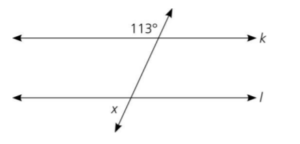# Euclidean GeometryTwo complimentary angles in Euclidean geometry.Euclidean geometry is the familiar geometry you’re taught in school: angles in a triangle always add up to 180°; a line has length but no width; a quadrilateral has four sides. It includes the Pythagorean theorem, the acronym SOHCAHTOA and ways to calculate the circumference and area of a circle. The word geometry comes from the Greek geometrein (“earth measure”). Euclidean geometry is named after the Greek mathematician Euclid (330-270 B.C.), who first described it. It’s called Euclidean geometry to differentiate it from other types of geometry, like taxicab geometry. Of all the existing geometries, Euclidean geometry is the only one to satisfy the fifth postulate, which reads:

“If two lines in the same plane are cut by a transversal so that the sum of the measures of a pair of interior angles on the same side of the transversal is less than 180, the lines will meet on that side of the transversal” (Wang, 2021).

Any geometry which doesn’t meet this postulate is called non-Euclidean.

## Origins of Euclidean Geometry

The origins of many geometric relationships like congruency and similarity can be traced back to the late stone age (Byer, 2010). Although many early works in the field were lost to history, one very significant text survived— Elements of Geometry by Euclid, which contains all the geometrical knowledge known at the time: 467 propositions on the geometry of planes and solids. Euclid’s Elements, as it came to be known, was the go-to text for over 2,000 years. It is only in recent years that other, non-Euclidean geometries have been discovered.

## References

Byer, O. et al. (2010). Methods for Euclidean Geometry. The Mathematical Association of America. Wang, Y. (2021). Chapter 4: Euclidean Geometry. Retrieved January 4, 2021 from: http://www2.fairmontstate.edu/users/ywang/teaching/fsu/courses/geometry_372/lecture_372_ch4.pdf

CITE THIS AS:
Stephanie Glen. "Euclidean Geometry" From StatisticsHowTo.com: Elementary Statistics for the rest of us! https://www.statisticshowto.com/euclidean-geometry/